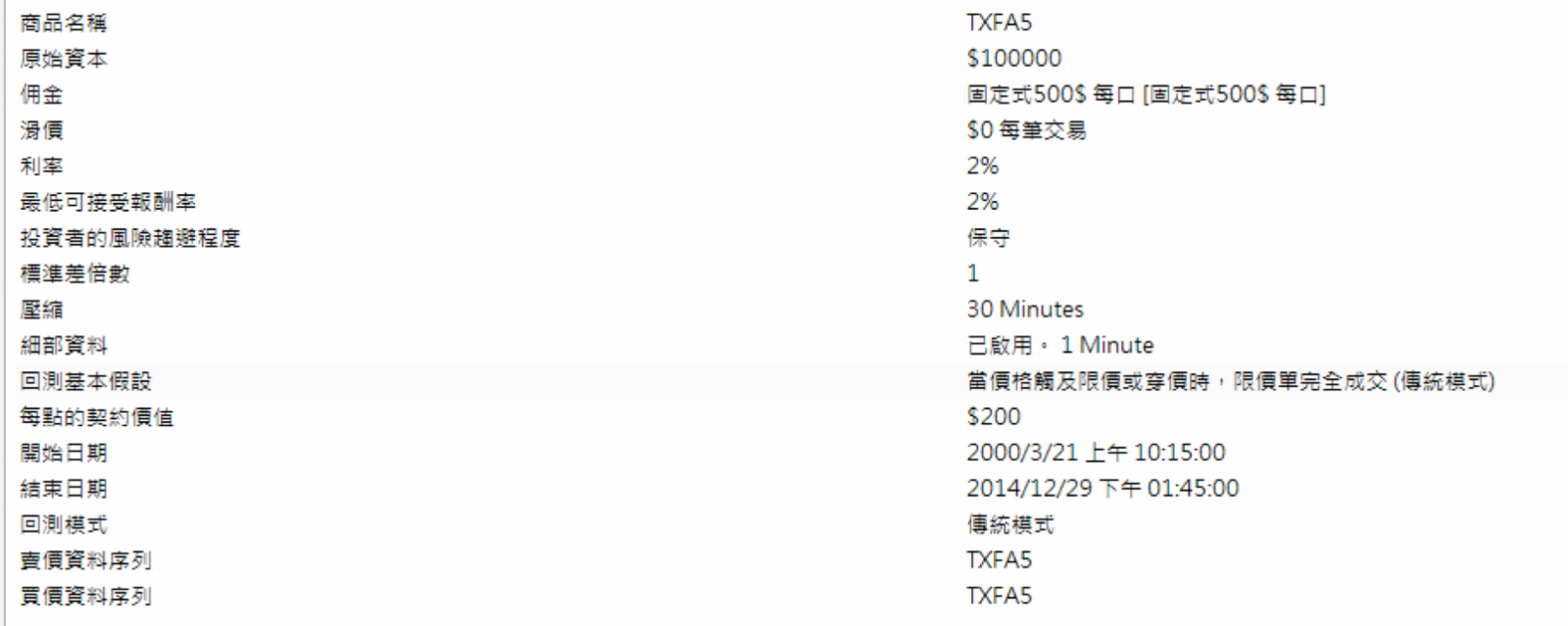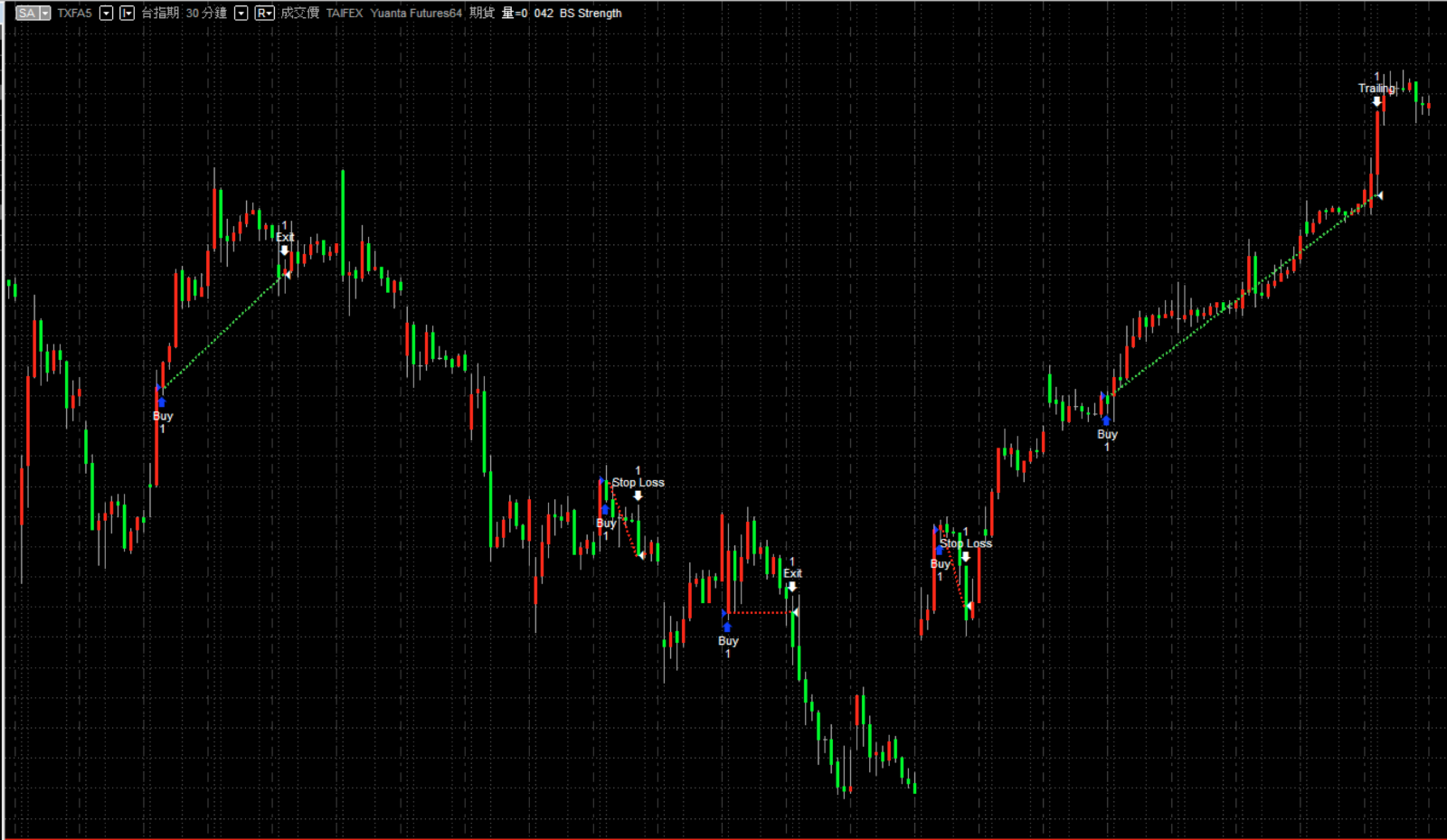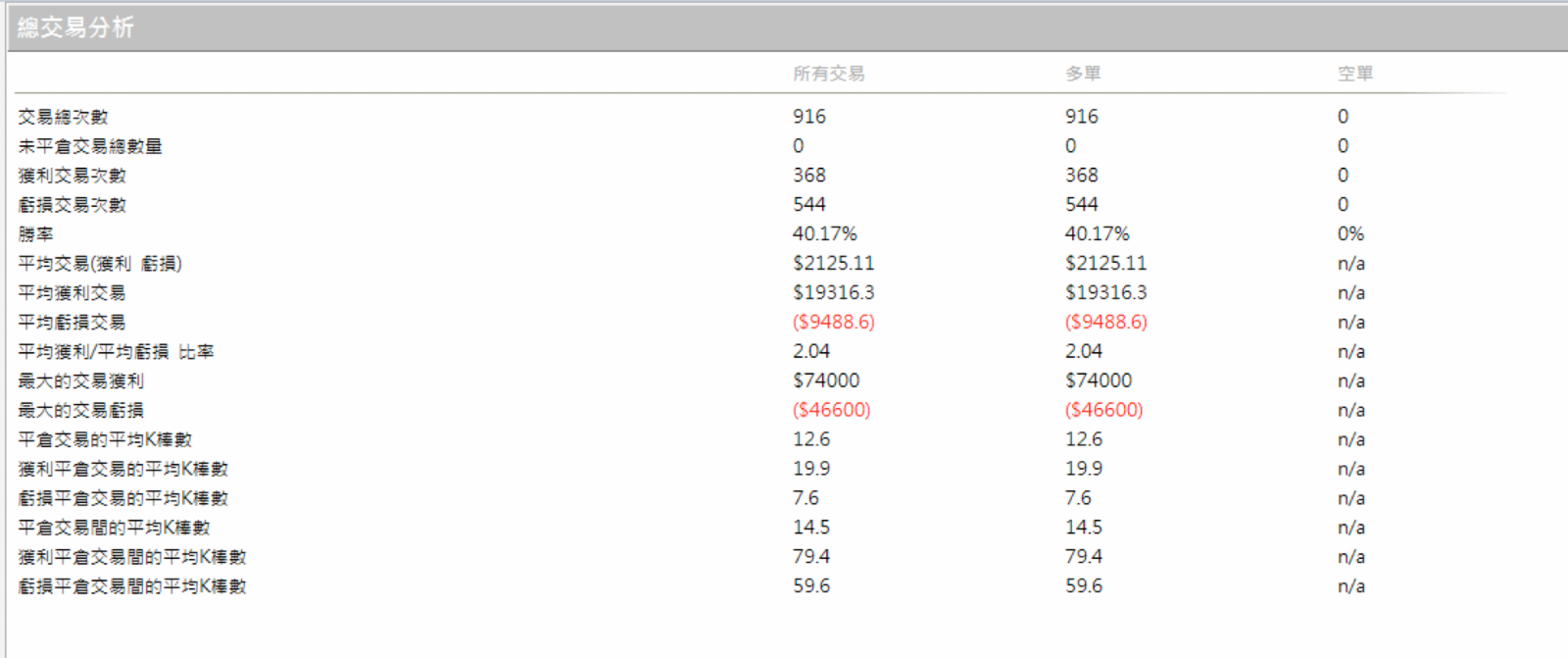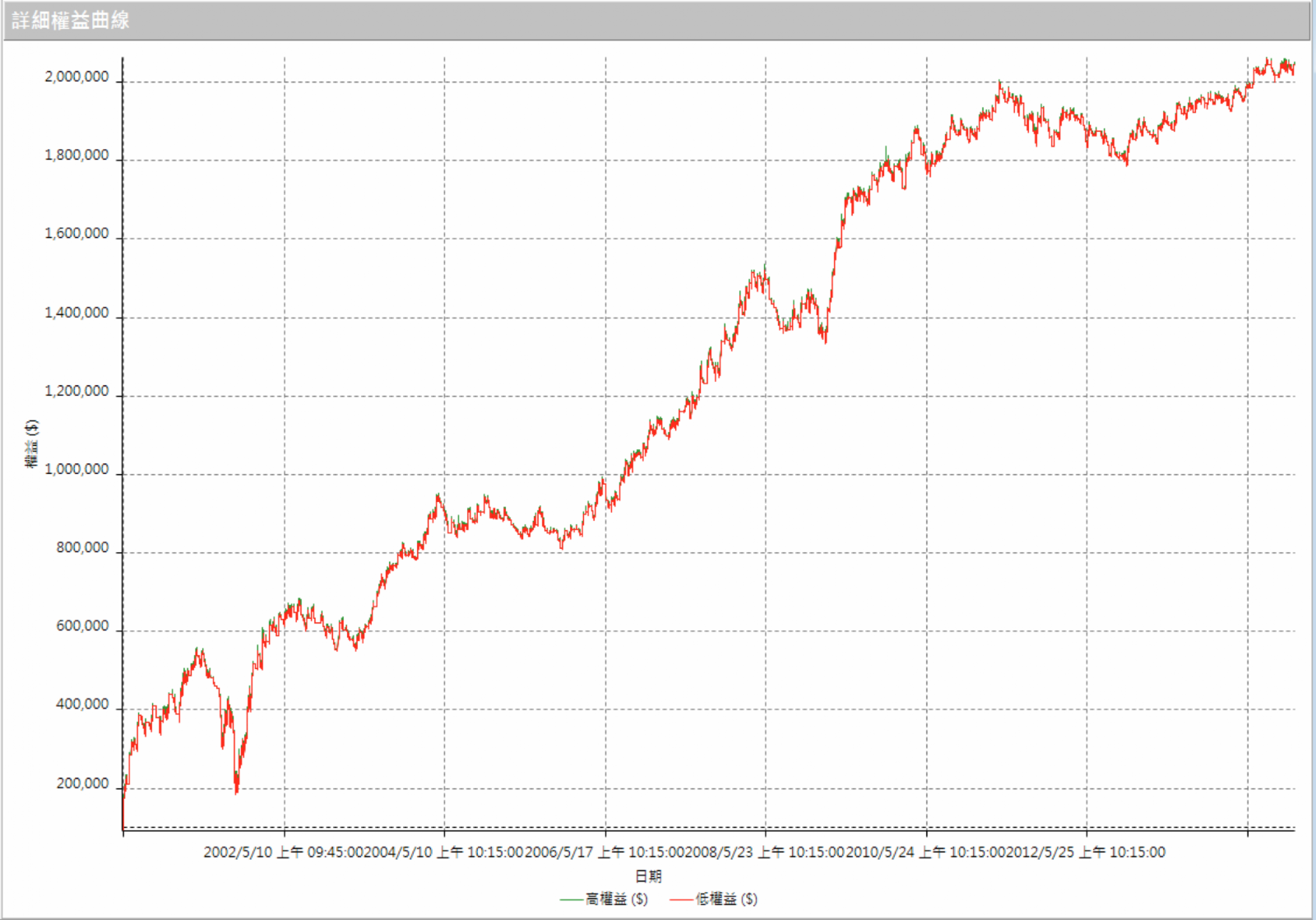## 2014年12月31日 星期三

### ★《大漲的訊號》買賣力道比例策略應用於台指期

《Wen外期策略團隊》If c>c then begin
B1=absvalue(o-c);
B2=absvalue(h-l);
S1=absvalue(o-l);
S2=absvalue(h-c);
end
else begin
B1=absvalue(h-o);
B2=absvalue(c-l);
S1=absvalue(c-o);
S2=absvalue(h-l);
end;

if (B1+B2+S1+S2)<>0 then BuyStrength=Round(Ticks*(B1+B2)/(B1+B2+S1+S2),0);
if (B1+B2+S1+S2)<>0 then ShortStrength=Round(Ticks*(S1+S2)/(B1+B2+S1+S2),0);

ShortTotal=Summation(ShortStrength,length);

#### 張貼留言

----------------------------------------------------------------------------------------------------

-------------------------------------------------------------------------------------------------